S.M. Nikol’skii Mathematical Institute
S.M. Nikol’skii Mathematical Institute
S.M. Nikol’skii Mathematical Institute conducts research in the field of functional analysis, theory of functional spaces, ordinary differential equations, partial differential equations, nonlinear analysis, spectral theory of differential operators.Director
Alexander Skubachevskii
Contacts
News All news
Science
27 Feb
RUDN University agrochemist visualised how plants get phosphorus from the soil

RUDN University soil scientists were able to visualise for the first time the activity of the phosphatase enzyme in the soil surrounding the roots of a plant by simultaneously using zymography and a fibre optic acidity sensor. This helped them to see how the plants “adjust” their root system to the environment. The study was published in the journal Soil Biology and Biochemistry.

Science
14 Feb
RUDN University bioengineers have created nanocontainers for targeted drug delivery

RUDN University bioengineers have created magnetic nanocontainers for “smart” delivery of drugs in the body. Thanks to them, the active substance enters only certain organs or tissues, which reduces the risk of side effects. In an experiment on mice, it was shown that nanocontainers are non-toxic and not dangerous. The results of the study are published in the journal Polymers.

Science
14 Feb
RUDN University chemist creates a catalyst to remove paracetamol from wastewater

A RUDN University chemist has created a catalyst for wastewater treatment that removes paracetamol – one of the most commonly prescribed antipyretics. This medicine is excreted from the body through the kidneys and enters the sewage, where it does not decompose and can pose a threat to people and the environment. The chemist managed to create a catalyst based on silver sulfide, zinc oxide, and graphene oxide to decompose paracetamol under light irradiation. The study is published in the journal Separation and Purification Technology.

Main areas of research All areas
Research projects All projects
Many problems that are studied in mathematical analysis can be reformulated in terms of the action of various operators in function spaces.

Construction of spherically symmetric stationary solutions of the Vlasov-Poisson system of equations describing the stationary distribution of particles in a gravitational field. Obtaining sufficient conditions for confining high-temperature plasma in a “mirror-trap” fusion reactor.

In the problem of describing the asymptotic properties of generalized solutions of quasilinear parabolic equations in a neighborhood of the time of the singular exacerbation of the boundary regime (i.e. boundary data), at the present time, it were found limiting restrictions on the intensity of the exacerbation leading to solutions with a non zero but finite measure of the blow- up, i.e. the so-called S-modes are described.

Laboratories and centers All laboratories

The laboratory is a structural subdivision of the Mathematical Institute. S. M. Nikolsky specializes in the field of mathematical modeling.

The center is a structural subdivision of the S.M. Nikol’skii Mathematical Institute specializes in the field of mathematical physics.

TypeCenter

Partners All partners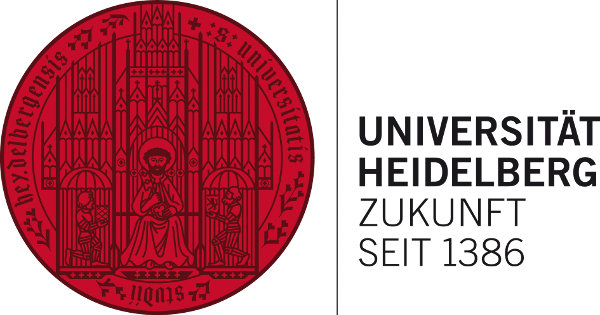City

Heidelberg, Germany

Type of institution

University

Subject of cooperation:
Conducting joint research in the field of automatic thermal control with hysteresis, building mathematical models of thermal control processes occurring in chemical reactors and climate control systems, research in the field of mathematical medicine. Publication of joint works in high-rated journals.
Start of cooperation: 2007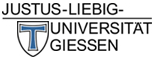City

Giessen, Germany

Type of institution

University

Subject of cooperation:
Conducting joint research in the field of nonlinear functional-differential equations. Publication of joint works in high-rated journals.
Result of cooperation:

Joint research work with H.-O.-Walther, an employee of Justus Liebig University. Sufficient terms of hyperbolicity and stability of periodic solutions of nonlinear functional-differential equations were obtained. The results of the work are reflected in the articles: - Walter H.-O., Skubachevskii A. L. On hyperbolicity of rapidly oscillating periodic solutions to functional differential equations. // Journal “Functional analysis and its applications”, Vol. 39, is. 1, M., 2005, p. 82-85. - Walter X-Skubachevskii A. L. On hyperbolicity of solutions with irrational periods of some functional differential equations. // Journal “Proceedings of the Russian Higher School Academy of Sciences”, Vol. 402. №2, M., 2005, p. 151-154.

Start of cooperation: 2003
Field of cooperation: hyperbolicity of periodic solutions of nonlinear functional-differential equations.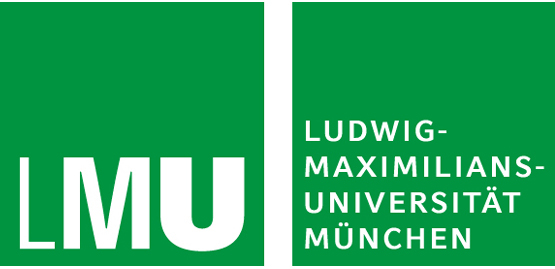City

Munich, Germany

Type of institution

University

Subject of cooperation:
- Conducting joint research in the field of stationary solutions of the Vlasov-Poisson system describing the distribution of particles in the gravitational field.
- Publication of joint works in high-rated journals
Result of cooperation:

We are working on the joint article “Spherical symmetric stationary solutions of the Vlasov – Poisson equation” with J. Batt, an employee of Ludwig Maximilian University of Munich.

Start of cooperation: 2017.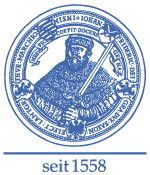City

Jena, Germany

Type of institution

University

Subject of cooperation:
- Conducting joint research in the field of optimal embedding, estimates of continuity modules.
- Publication of joint works in high-rated journals.
Result of cooperation:

Joint research work with D. Haroske, an employee of Friedrich Schiller University Jena. Evaluation of the uniform modulus of continuity for Bessel potentials, accurate evaluation of the majorant of modules of continuity and optimal embedding for generalized Bessel potentials were obtained, optimal Calderon space for Bessel potentials and optimal Calderon space for generalized Bessel potentials were built.

Start of cooperation: 2013.City

Hannover, Germany

Type of institution

University

Subject of cooperation:
- Conducting joint research in the field of the theory of elliptic operators, noncommutative geometry.
- Publication of joint papers in high-rated journals
Result of cooperation:

Mathematicians of RUDN University (Professor B. Yu. Sternin, Professor A. Y. Savin) with mathematicians of the University of Hannover (Prof. Dr. Elmar Schrohe) analyze actual problems of elliptic theory and noncommutative geometry in joint scientific works. In particular, an explicit uniformization for elliptic operators associated with groups of shift operators was found out. The terms for the Fredholm property of the operators associated with groups of quantized canonical transformations were obtained.

A.Yu. Savin, B.Yu. Sternin, E. Schrohe, “Index problem for elliptic operators associated with a diffeomorphism of a manifold and uniformization”, Proceedings of the Russian Higher School Academy of Sciences, 441:5 (2011), 593–596; A.Yu. Savin, B.Yu. Sternin, E. Schrohe, “Index problem for elliptic operators associated with a diffeomorphism of a manifold and uniformization”, Dokl. Math., 84:3 (2011), 846-849

A.Yu. Savin, B.Yu. Sternin, E. Schrohe, “On the Index Formula for an Isometric Diffeomorphism”, Journal of Mathematical Sciences, 201:6 (2014), 818–829

A.Yu. Savin, E. Schrohe, B. Sternin, “Uniformization and Index of Elliptic Operators Associated with Diffeomorphisms of a Manifold”, Russian Journal of Mathematical Physics, 22:3 (2015), “410–420”

Start of cooperation: 2011.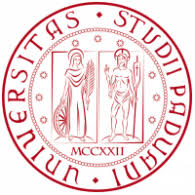City

Type of institution

University

Subject of cooperation:
- Conducting joint research in the field of spectral theory.
- Publication of joint works in high-rated journals.
Result of cooperation:

Accurate stability evaluation for the variation of eigenvalues of nonnegative selfadjoint elliptic operators of arbitrary even order when changing the open sets on which they are defined were obtain with P. D. Lamberti, M. Lanza de Cristoforis, Feleqi E. This evaluation is expressed in terms of Lebesgue measure of the symmetric difference of open sets. The boundary terms of Dirichlet and Neumann were analyzed.

Start of cooperation: 2006.
Field of cooperation: spectral theory.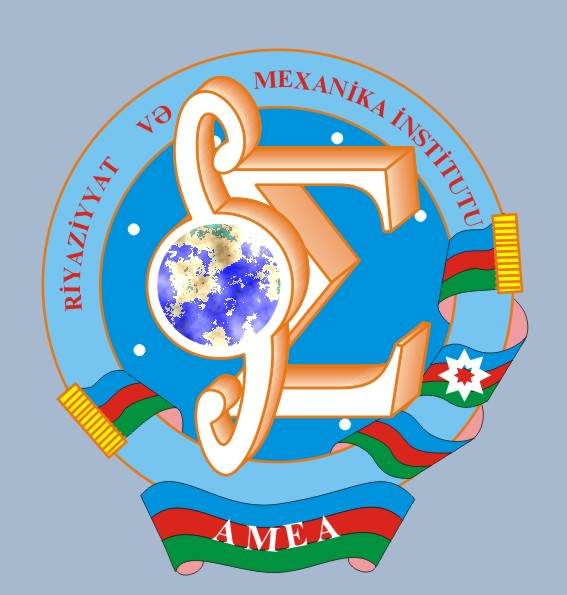City

Baku, Azerbaijan

Type of institution

Research Institute

Subject of cooperation:
- Conducting joint research in the field of operator theory in Morrie type spaces.
- Publication of joint works in high-rated journals.
Start of cooperation: 2006.
Field of cooperation: theory of operators in Morrie type spaces.City

Ramat-Gan, Israel

Type of institution

University

Subject of cooperation:
- Conducting joint research in the field of operator theory in Morrie type spaces.
- Publication of joint works in high-rated journals.
Result of cooperation:

The terms that ensure the boundedness of Hausdorff operators in Morrie spaces were obtained together with E. Liflyand. The classes of Hausdorff operators for which the necessary and sufficient terms of boundedness coincide were described.

Start of cooperation: 2017.City

Praga, Czech Republic

Type of institution

Research Institute

Subject of cooperation:
- Conducting joint research in the field of operator theory in Morrie type spaces.
- Publication of joint works in high-rated journals.
Result of cooperation:

Necessary and sufficient terms for the boundedness of fractional maximal operators in general Morrie local spaces are for a wide class of admissible values of numerical parameters were obtained together with A. Gogatishvili. Sufficient and necessary terms for this kind of limitation for a certain range of parameters were obtained.

Start of cooperation: 2010.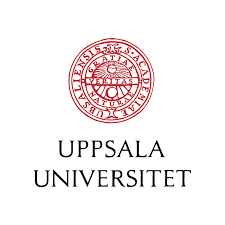City

Uppsala, Sweden

Type of institution

University

Subject of cooperation:
Development of new modeling methods in Biomedicine based on discrete-continuous approaches in which biological cells are considered as individual objects, intracellular regulation is modeled by ordinary differential equations, and intercellular concentrations were described by partial differential equations. Application of these methods to modeling of various physiological processes.
Result of cooperation:

Joint research work with A. Bouchnita. Mathematical models and computer programs for describing the coagulation of blood in the stream considering biochemical reactions in the plasma and aggregation of platelets were developed. The terms of normal clot growth and thrombosis were obtained. Two articles are in print this year.

Start of cooperation: 2018.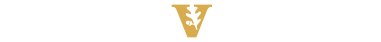City

Nashville, USA

Type of institution

University

Subject of cooperation:
Mathematical modeling of erythropoiesis and blood cancers such as multiple myeloma. Development of optimal chemotherapy methods for patients with multiple myeloma.
Result of cooperation:

Joint research work with M. Koury. The mathematical model was developed, computer calculations and comparisons with experimental data of erythropoiesis functioning on the basis of erythroblastic islands were carried out. The development of multiple myeloma and its effect on erythropoiesis were investigated. Various treatment protocols of patients were modeled and compared with clinical data.

Start of cooperation: 2012.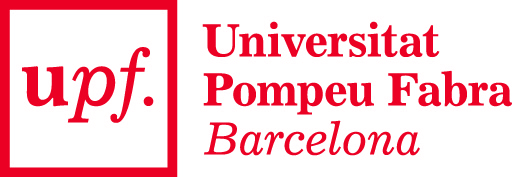City

Barcelona, Spain

Type of institution

University

Subject of cooperation:
Multiscale and spatial models of immune response.
Result of cooperation:

Joint research work with A. Meyerhans. The study of the spread of viral infection in body tissues on the basis of reaction-diffusion and hybrid discrete-continuous models of immune response.

Start of cooperation: 2016.City

Lyon, France

Type of institution

University

Subject of cooperation:
Mathematical modeling of various diseases and clinical trials on virtual patients.
Result of cooperation:

Joint research work with C. Dumontet, P. Nony. Mathematical modeling of variousdiseases: leukaemia, lymphoma, lymphodema, mucoviscidosis, thrombosis. Simulation of clinical trials.

Start of cooperation: 2012.City

St. Gallen, Switzerland

Type of institution

Research Centre

Subject of cooperation:
Joint research with Prof. Ludwig Burkhardt's group on “Mathematical modeling of spatial structure and functioning of lymphoid organs”.
Result of cooperation:

Parametric models of the geometry of the lymph node were developed. Models were developed and topological properties of a network of fibroblastic reticular cells were analyzed. The features of lymph flow in the network of lymph node conduits were studied.

Start of cooperation: 2000.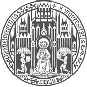City

Heidelberg, Germany

Type of institution

Research Centre

Subject of cooperation:
Joint research with Prof. Willy Yeager on mathematical modeling of sepsis.
Result of cooperation:

The components of the system model describing the regulation of energy metabolism of cells in normoxia and hypoxia were developed.

Start of cooperation: 2015.
Events All events
2020
12 Mar
Scientific seminar “Geometric combinatorics, commutative algebra and algebraic topology-V”
In the framework of the seminar, we will consider the Cohen-Macaulay and shelling simplicial complexes, study the construction of the Stanley-Reisner ring, and also formulate the theorems of Hochster (without proof), Reisner and Mankrs (with outline of the proofs).
2020
5 Mar
Seminar on mathematical modeling in biology and medicine “Neural field models and brain stimulation”
We will discuss neural field models and wave propagation in the brain cortex.
2020
5 Mar
Postgraduate Mathematical Seminar “The fundamental theorem of calculus for Banach space-valued functions”
Topic: The fundamental theorem of calculus for Banach space-valued functions